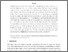# Weighted scores estimating equations and CL1 information criteria for longitudinal ordinal response

Nikoloulopoulos, Aristidis KORCID: https://orcid.org/0000-0003-0853-0084 (2020) Weighted scores estimating equations and CL1 information criteria for longitudinal ordinal response. Journal of Statistical Computation and Simulation, 90 (11). pp. 2002-2022. ISSN 0094-9655Preview PDF (wtsc-ord) - Accepted Version Download (257kB) | Preview

## Abstract

Available extensions of generalized estimating equations for longitudinal ordinal response require a conversion of the ordinal response to a vector of binary category indicators. That leads to a rather complicated working correlation structure and to large matrices when the number of categories and dimension of the clusters are large. Weighted scores estimating equations are constructed to overcome the aforementioned problems. Similar to generalized estimating equations which construct unbiased equations weighting the residuals, the weighted scores weight the univariate score functions. To specify the weight matrices, the weighted scores estimating equations use a working dependence model, namely the multivariate normal (MVN) copula model with univariate ordinal probit or logit regressions as the marginals. There is no need to convert the ordinal response to binary indicators, thus the weight matrices have smaller dimensions. Composite likelihood information criteria are further proposed as an intermediate step for selecting both the covariates in the mean function modelling and the structure of the latent correlation matrix induced by the MVN latent variables. The weighted scores estimating equations and composite likelihood information criteria are illustrated by analyzing a rheumatoid arthritis clinical trial. Our modelling framework is implemented in the package weightedScores within the open source statistical environment R.

Item Type: Article arXiv preprint bic,composite likelihood,correlation structure selection,generalized estimating equations,ordinal regression,variable selection,statistics and probability,modelling and simulation,statistics, probability and uncertainty,applied mathematics ,/dk/atira/pure/subjectarea/asjc/2600/2613 Faculty of Science > School of Computing Sciences Faculty of Science > Research Groups > Data Science and Statistics http://www.scopus.com/inward/record.url?... LivePure Connector 14 May 2020 23:59 22 Oct 2022 00:22 https://ueaeprints.uea.ac.uk/id/eprint/75162 10.1080/00949655.2020.1759602View Item